| Next|| Previous || Top |

# SUMMARY OF DATA INPUT

## ADVECTION

-cells cells

-shifts shifts

-time_step time step

-initial_time initial_time

-print_cells list of cell numbers

-print_frequency print_modulus

-punch_cells list of cell numbers

-punch_frequency punch_modulus

-warnings [( True or False )]

## EQUILIBRIUM_PHASES

EQUILIBRIUM_PHASES [ number ] [ description ]

phase name [ saturation index [( alternative formula or alternative phase ) [ amount ]]]

## EXCHANGE

EXCHANGE [ number ] [ description ]

exchange formula, amount

exchange formula, name, [( equilibrium_phase or kinetic_reactant)] , exchange_per_mole

-equilibrate number

## EXCHANGE_MASTER_SPECIES

exchange name, exchange master species

## EXCHANGE_SPECIES

Association reaction

log_k log K

delta_h enthalpy, [ units ]

-analytical_expression A 1 , A 2 , A 3 , A 4 , A 5

-gamma Debye-Hückel a, Debye-Hückel b

-davies

-no_check

-mole_balance formula

## GAS_PHASE

### Fixed-pressure gas phase

GAS_PHASE [ number ] [ description ]

-fixed_pressure

-pressure pressure

-volume volume

-temperature temp

phase name, partial pressure

### Fixed-volume gas phase: Define initial moles of components with partial pressures

GAS_PHASE [ number ] [ description ]

-fixed_volume

-volume volume

-temperature temp

phase name, partial pressure

### Fixed-volume gas phase: Define initial moles of components by equilibrium with a solution

GAS_PHASE [ number ] [ description ]

-fixed_volume

-equilibrium number

-volume volume

phase name

## INCREMENTAL_REACTIONS

INCREMENTAL_REACTIONS [( True or False )]

## INVERSE_MODELING

INVERSE_MODELING [ number ] [ description ]

-solutions list of solution numbers

-uncertainty list of uncertainty limits

-phases

phase name [ force] [ dissolve or precipitate] [ list of isotope name, isotope ratio, isotope uncertainty limit ]

-balances

element or valence state name, [ list of uncertainty limits ]

-isotopes

isotope_name, list of uncertainty limits

-range [ maximum ]

-minimal

-tolerance tol

-force_solutions list of ( True or False )

-uncertainty_water moles

-mineral_water [( True or False )]

## KINETICS

### Explicit definition of steps

KINETICS [ number ] [ description ]

rate name

-formula list of formula, [ stoichiometric coefficient ]

-m moles

-m0 initial moles

-parms list of parameters

-tol tolerance

-steps list of time steps

-step_divide step_divide

-runge_kutta ( 1, 2, 3, or 6)

### Equal-increment definition of steps

-steps total time [ in steps ]

## KNOBS

-iterations iterations

-convergence_tolerance convergence_tolerance

-tolerance tolerance

-step_size step_size

-pe_step_size pe_step_size

-diagonal_scale [( True or False )]

-debug_diffuse_layer [( True or False )]

-debug_inverse [( True or False )]

-debug_model [( True or False )]

-debug_prep [( True or False )]

-debug_set [( True or False )]

-logfile [( True or False )]

## MIX

MIX [ number ] [ description ]

solution number, mixing fraction

## PHASES

Phase name

Dissolution reaction

log_k log K

delta_h enthalpy, [ units ]

-analytical_expression A 1 , A 2 , A 3 , A 4 , A 5

-no_check

## PRINT

-reset [( True or False )]

-eh [( True or False )]

-equilibrium_phases [( True or False )]

-exchange [( True or False )]

-gas_phase [( True or False )]

-headings [( True or False )]

-inverse_modeling [( True or False )]

-kinetics [( True or False )]

-other [( True or False )]

-saturation_indices [( True or False )]

-solid_solutions [( True or False )]

-species [( True or False )]

-surface [( True or False )]

-totals [( True or False )]

-user_print [( True or False )]

-selected_output [( True or False )]

-status [( True or False )]

## RATES

name of rate expression

-start

numbered Basic statements

-end

## REACTION

### Explicit definition of steps

REACTION [ number ] [ description ]

( phase name or formula ), [ relative stoichiometry ]

list of reaction amounts, [ units ]

### Equal increment definition of steps

reaction amount [ units ] [ in steps ]

## REACTION_TEMPERATURE

### Explicit definition of steps

REACTION_TEMPERATURE [ number ] [ description ]

list of temperatures

### Equal increment definition of steps

temp 1 , temp 2 , in steps

## SAVE

SAVE keyword , number

## SELECTED_OUTPUT

-file file name

-selected_out [( True or False )]

-user_punch [( True or False )]

-high_precision [( True or False )]

-reset [( True or False )]

-simulation [( True or False )]

-state [( True or False )]

-solution [( True or False )]

-distance [( True or False )]

-time [( True or False )]

-step [( True or False )]

-pH [( True or False )]

-pe [( True or False )]

-reaction [( True or False )]

-temperature [( True or False )]

-alkalinity [( True or False )]

-ionic_strength [( True or False )]

-water [( True or False )]

-charge_balance [( True or False )]

-percent_error [( True or False )]

-totals element list

-molalities species list

-activities species list

-equilibrium_phases phase list

-saturation_indices phase list

-gases gas-component list

-kinetic_reactants reactant list

-solid_solutions component list

-inverse_modeling [( True or False )]

## SOLID_SOLUTIONS

SOLID_SOLUTIONS [ number ] [ description ]

solid-solution name

-comp phase name, moles

-comp1 phase name, moles

-comp2 phase name, moles

-temp temperature in Celsius

-tempk temperature in Kelvin

-Gugg_nondim a0, a1

-Gugg_kJ a0, a1

-activity_coefficients,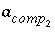, x 1 , x 2

-distribution_coefficients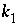,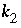, x 1 , x 2

-miscibility_gap x 1 , x 2

-spinodal_gap x 1 , x 2

-critical_point x cp , t cp

-alyotropic_point x aly ,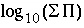-Thompson wg 2 , wg 1

-Margules alpha 2 , alpha 3

## SOLUTION

SOLUTION [ number ] [ description ]

temp temperature

pH pH [( charge or phase name [ saturation index ])]

pe pe [( charge or phase name [ saturation index ])]

redox redox couple

units concentration units

density density

element list, concentration, [ units ], ([ as formula ] or [ gfw gfw ]), [ redox couple ], [( charge or phase name [ saturation index ])]

-isotope name , value, [ uncertainty limit ]

-water mass

## SOLUTION_MASTER_SPECIES

element name, master species, alkalinity, ( gram formula weight or formula ) , gram formula weight of element

## SOLUTION_SPECIES

Association reaction

log_k log K

delta_h enthalpy, [ units ]

-analytical_expression A 1 , A 2 , A 3 , A 4 , A 5

-gamma Debye-Hückel a, Debye-Hückel b

-no_check

-mole_balance formula

## SOLUTION_SPREAD

-temp temperature

-pH pH

-pe pe

-redox redox couple

-units concentration units

-density density

-water mass

-isotope name, value, [ uncertainty_limit ]

-isotope_uncertainty name, uncertainty_limit

column headings

[ subheadings ]

chemical data

## SURFACE

### Implicit definition of surface composition

SURFACE [ number ] [ description ]

-equilibrate number

surface binding-site name, sites, specific_area_per_gram, mass

surface binding-site formula, name, [( equilibrium_phase or kinetic_reactant)] , sites_per_mole, specific_area_per_mole

-no_edl

-diffuse_layer [ thickness ]

-only_counter_ions

### Explicit definition of surface composition

SURFACE [ number ] [ description ]

surface binding-site formula, sites, specific_area_per_gram, mass

surface binding-site formula, name, [( equilibrium_phase or kinetic_reactant)] , sites_per_mole, specific_area_per_mole

## SURFACE_MASTER_SPECIES

surface binding-site name, surface master species

## SURFACE_SPECIES

Association reaction

log_k log K

delta_h enthalpy, [ units ]

-analytical_expression A 1 , A 2 , A 3 , A 4 , A 5

-no_check

-mole_balance formula

TITLE comment

comment

## TRANSPORT

-cells cells

-shifts shifts

-time_step time step

-flow_direction ( forward, back, or diffusion_only)

-boundary_conditions first, last

-lengths list of lengths

-dispersivities list of dispersivities

-correct_disp [( True or False )]

-diffusion_coefficient diffusion coefficient

-stagnant stagnant_cells [ exchange_factor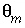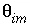]

-thermal_diffusion temperature retardation factor, thermal diffusion coefficient

-initial_time initial_time

-print_cells list of cell numbers

-print_frequency print_modulus

-punch_cells list of cell numbers

-punch_frequency punch_modulus

-dump dump file

-dump_frequency dump_modulus

-dump_restart shift number

-warnings [( True or False )]

## USE

USE keyword , ( number or none)

## USER_PRINT

-start

numbered Basic statements

-end

## USER_PUNCH

-headings list of column headings

-start

numbered Basic statements

-end

| Next|| Previous || Top |